metamerist

Wednesday, August 30, 2006

Linear Algebra for Graphics Geeks (SVD-V)

This is post #5 of Linear Algebra for Graphics Geeks. Here are links to posts #1, #2, #3, #4. The subject at hand is the Singular Value Decomposition.

Let's look at the equation for the SVD again:

A = U*S*V'

We've already seen how to determine if A is singular by examining the diagonal of S. Now we'll consider the subject of matrix inversion.

One rule of linear algebra will help us here:

The inverse of a matrix product is the same as the reverse product of matrix inverses. (Mathworld: Matrix Inverse)

E.g.,

(ABC)-1 = C-1B-1A-1

Given this rule and A = U*S*V', we have the following:

A-1 = (U*S*V')-1 = V'-1*S-1*U-1

In part #2, we discovered U and V are orthogonal matrices. Because of this, their transposes and their inverses are one and the same. Given that knowledge, we can simplify our inverse equation.

A-1 = (V')-1*S-1*U-1 = V*S-1*U'

(See how easy it is to manipulate the SVD!)

Now all we need to do is figure out how to invert S, which is easy because S is a diagonal matrix (a.k.a. scale matrix). Consider a simple 2x2 scale matrix that scales X by 2 and Y by 3:

[2 0]
[0 3]

What's the inverse of this matrix? It's the matrix that scales X by 1/2 and Y by 1/3:

[1/2 0]
[ 0 1/3]

If we multiply these two together, we get the identity matrix.

[2 0]*[1/2 0] = [2*1/2 0] = [1 0]
[0 3]*[0 1/3] . [0 3*1/3] . [0 1]

The inverse of a diagonal matrix is calculated by replacing each element on the diagonal with its reciprocal.

This makes perfect sense. We covered inversion in one dimension in part #3 with our discussion of inches and centimeters. Naturally this extends to multiple dimensions; e.g., scaling width, height, depth all involve multiplying by some scalar, and reversing the scaling requires multiplication by the inverses of those scalars.

Of course, if any element on the diagonal of S is zero, we will wind up trying to divide by zero when we attempt to invert S; when that happens, it's because A is singular (and therefore S is singular #3). Also, if S is almost singular, some of the elements on the diagonal will be very tiny numbers and their reciprocals will be very huge numbers. In such a case, our inverse may not be very useful.

Next, I discuss eigenvectors, eigenvalues and ways in which they are related to the SVD.

Tuesday, August 29, 2006

Linear Algebra for Graphics Geeks (SVD-IV)

This is post #4 of Linear Algebra for Graphics Geeks. Here are links to posts #1, #2, #3. The subject at hand is the Singular Value Decomposition.

In the last episode we looked at the determinant and its relationship to the SVD. In closing we came to the realization that zeroes on the diagonal of S were evidence of A's singularity.

While a zero determinant indicates the singularity of a matrix (it has no inverse), a really great property of the SVD is that it not only tells you if a matrix is singular, but also gives you an indication of which dimensions have been blown away (zeroed).

Let's look at a very simple example. Consider the following 2x2 matrix.

[1 0 ]
[0 0]

Transforming 2D vectors [x y]' with this matrix eliminates the y component, projecting everything down onto the x axis. That is,

new_x = [1 0] * [x y]'
new_y = [0 0] * [x y]'

No matter what, the new transformed value of y becomes zero. The SVD of this matrix is (U*S*V'). Ignore the periods--I'm just trying to maintain spacing in Blogger.

[1 0] * [1 0] * [1 0]
[0 1] . [0 0] . [0 1]

Note that U and V' (and V) are equal to the identity matrix. The first column of U is [1 0], which is a unit vector along the x axis. The second column of U is [0 1], which is a unit vector along the y axis.

The top-left element of S is 1, which shows that the dimension indicated by the first column of U (in this case, the x axis) is unchanged (scaled by 1); the bottom-right element of S is 0, which indicates the dimension indicated by the second column of U (the y axis) is blown away to zero.

Let's look at another matrix.

[2 0]
[0 0]

Like the first example, this matrix again zeroes y values, but this time the scaling factor for the x dimension is 2, so all x values are doubled. The SVD of this matrix is (U,S,V').

[1 0] * [2 0] * [1 0]
[0 1] . [0 0] . [0 1]

The only change from the previous result is the top-left element of S has changed to 2, indicating the new scaling factor for the x dimension.

This time, let's eliminate the x dimension and double the y dimension.

[0 0 ]
[0 2 ]

The SVD for this matrix (U,S,V') is:

[0 1] * [2 0] * [0 1]
[1 0] . [0 0] . [1 0]

It might be surprising that S is the same as it was in the previous example. Why? We're not scaling the x dimension by 2 this time--we're scaling y by 2. Why is the top-left of S still 2? The answer: The SVD sorts the elements on the diagonal of S in descending order. The top-left element of S contains that largest value and the bottom-right element of S contains the smallest value (we're still assuming square matrices).

If you look at U, you'll see that the column vectors have been swapped. The first column vector [0 1] is a unit vector on the y axis. The second column vector of U is the unit vector [1 0] on the x axis. You'll also see the rows of V' have been swapped (although it's impossible to differentiate between a column swap and a row swap in this case, the rows of V' are our concern).

One reason these examples are so simple and clean is that there's no rotation involved. We've simply scaled the x and y axes without rotation. As a consequence, the orthonormal basis comprised by the columns of U consists of vectors on the x and y axes.

Here's another nice property of the SVD. Zeroes on the diagonal of S indicate which of the column vectors of U are singular. Not only that, values near zero on the diagonal of S indicate which of the column vectors of U are nearly singular. This is valuable information because near zero is often as bad as zero. Also, the rank is easily determined by inspecting S; the rank of A is equal to the number of non-zero elements on the diagonal of S.

Next, let's look at matrix inversion.

Sunday, August 27, 2006

Begin to HopeI've mentioned Regina Spektor a couple of times (1,2). At this point, her new disc, Begin to Hope, has racked up a deserved 79 on Metacritic. Metamerist recommended! (I think her song Us from her disc Soviet Kitsch is one of the best pop songs I've heard in the past few years. It's on her site under Music.)

Also, I've been enjoying Zero 7's new disc, The Garden.To it's credit, it does have two marks of a winner: KCRW seems to like it and Pitchfork Media hates it. This sort of diametric opposition is always a good sign.

:)

Friday, August 25, 2006

Linear Algebra for Graphics Geeks (SVD-III)

This is post #3 of Linear Algebra for Graphics Geeks. Here are links to posts #1 and #2. The subject at hand is the Singular Value Decomposition.

Determinants & SVD

I remember learning determinants as being some sort of magical numbers that told you when a matrix was invertible. Then one day I stumbled onto a statement such as this:

"The fundamental geometric meaning of a determinant is as the scale factor for volume when A is regarded as a linear transformation." (Wikipedia)

Another way I like to say it is that the absolute value of the determinant is the volume of a unit cube after it's transformed by A. (In 2D, it's the area of a transformed unit square.)

Since rotations don't change volume in any way and determinants are scaling factors for volume, we can correctly infer that the absolute value of the determinant of a rotation matrix is 1. The determinant of an orthogonal matrix is always 1 or -1.

Note: I've read a lot of literature that discusses U*S*V' as rotation*scale*rotation. At the same time, I've seen cases where det(U)=-1, which Mathworld refers to as rotoinversion or improper rotation, so perhaps some authors are a little loose with their definitions of rotation. In any case, it's always true to say that U and V are orthogonal matrices

One rule of determinants is that the product of determinants is the same as the determinant of a product. In other words:

det(A) = det(U*S*V') = det(U)*det(S)*det(V')

Since U and V are orthogonal matrices with determinants of -1 or +1, the absolute value of the determinant of A must be the same as the absolute value of the determinant of S. Because S is a diagonal matrix, calculating its determinant is trivial.

The determinant of a diagonal matrix is simply the product of the diagonal elements (S(1,1) * S(2,2) * S(3,3) ... S(n,n)). Since we know determinant is "scale factor for volume," this makes perfect sense; e.g., the overall scale in 3D should be the product of the width scale * height scale * depth scale. One consequence of the SVD factoring A into U*S*V' is that all of the scaling winds up in the S matrix.

In linear algebra, determinants are primarily used to determine whether or not a matrix is invertible. If the determinant of a matrix is zero, the matrix is said to be singular. Trying to invert a singular matrix is the linear algebra equivalent of dividing by zero. Since I've seen people stumble in this area, I'm going to elaborate a bit.

Let's look at a one dimensional case.

When you convert from inches to centimeters, you scale everything up and multiply inches by a scaling factor of 2.54. When you want to go back to inches from centimeters, you multiply by the inverse 1/2.54. For every length you can can express in inches, from 0 to as big a number as you can imagine, there's an equivalent length in centimeters. Likewise, if you convert from feet to inches, you'll scale by 12 to go one direction and 1/12 to go the other.

As you know, there are many units of length, there are scaling factors used to convert between the various units, and for everyone, reversing a conversion involves multiplying by the inverse of the scaling factor used to do the original conversion. Everything works fine on one condition: the scaling factor can't be zero. If the scaling factor is zero, the result of the conversion, no matter which number you use, is always zero. 1*0=0, 2*0=0, 3*0=0, etc.

When you use zero as a scaling factor, you reduce a one dimensional space (length) down to a zero dimensional space (a point: zero). When that happens, there's no way to go back. You can try, but you'll wind up dividing by zero. You've lost a dimension, and there's no way to recover all of the lost information.

In the case of matrices as transformations, there are multiple ways to lose one or more dimensions. A matrix might transform everything in a 3D space down a single point, or a matrix might reduce everything in a 5D space down to a 2D plane. Whenever you lose a dimension or more, there's no way to map back, and when that's the case, we refer to the matrix as singular.

Let's think about something. We know that the determinant of a singular matrix is zero. We know that the determinant of an orthogonal matrix (U,V') is -1 or +1. We know that the product of determinants is the same as the determinant of products. This means that if the determinant of A is zero, it has to be due to something going on with S, because det(A) = det(U) * det(S) * det(V') and det(U) = det(V) = +/-1.

From another perspective, if matrix A blows away one or more dimensions, it's going to reduce the volume of everything it transforms down to zero. With the SVD all of the volume scaling is handled by the diagonal matrix S. The overall volume scaling factor (determinant) is the product of the elements on the diagonal of S. If one of the diagonal elements of S is zero, that means the determinant of S is zero and therefore the determinant of A is zero and therefore A is singular.

We're beginning to understand why this is called the Singular Value Decomposition. :)

Next, we look at simple cases involving matrix singularity.

Linear Algebra for Graphics Geeks (SVD-II)

This is post #2 of Linear Algebra for Graphics Geeks. The first post is here, and the subject at hand is the Singular Value Decomposition.

A = U*S*V'

Previously, we found the SVD factors a matrix into the product U*S*V' which amounts to a rotation * scale * rotation. From a linear algebra perspective, the rotation matrices U and V are orthogonal matrices and the scale matrix S is more commonly referred to as a diagonal matrix.

(Note: If A is not a square matrix, S will not be square, but that's something we'll need to address later. For now, let's assume A is square.)

Let's talk about U and V. Because U and V are orthogonal matrices, their inverses and their transposes are one and the same. (Orthogonal Matrix: Wikipedia, MathWorld). This makes it easy to manipulate the SVD equation. For example, let's multiply both sides of the equation by V.

A*V = U*S*V'*V

V' is the transpose of V. Because V is orthogonal, V' is also the inverse of V. Consequently, the product V'*V is the identity matrix and the V' and V on the right side of the equation cancel each other out leaving us with the following equation.

A*V = U*S

This equation also has interesting implications. U and V are orthogonal matrices, and another interesting property of orthogonal matrices is that the columns form an orthonormal basis (Wikipedia, Mathworld). What that means is if you consider each column of V to be a vector, you'll have a set of vectors that are all perpendicular to each other. (Note: the rows of an orthogonal matrix form an orthonormal basis as well, but let's focus on columns for now.)

In 2D, for example, the vectors [1 0] and [0 1] form an orthonormal basis. If you rotate them both together, they'll remain perpendicular but form a new orthonormal basis. If you think about it, the columns of a rotation matrix (Wikipedia, Mathworld) always form an orthonormal basis.

Back to our new equation...

A*V = U*S

Since the columns of V form an orthonormal basis and the columns of U form an orthonormal basis and S is a scale matrix, we have:

A * (orthonormal basis) = (orthonormal basis) * (scale matrix)

In other words, if we use the matrix A to transform a set of orthogonal vectors (the column vectors of V), our result is a new set of orthogonal vectors (the columns of U) multiplied by a scale matrix (S).

This is a point where visualizations can do a lot of good. Let's say V is an orthonormal basis in 2D. The rules apply in higher dimensions, but 2D is easy to visualize.

V might be [0 1] and [1 0] as we see below, or V might be any possible rotation of those two vectors. The space covered by sweeping through all possible rotations is the unit circle as seen below again.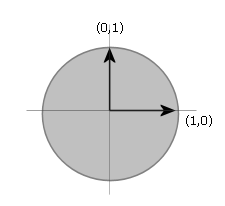If A is an invertible matrix (nonsingular), then A will transfer the unit circle into an ellipse. For example, A might transform the unit circle into the following ellipse.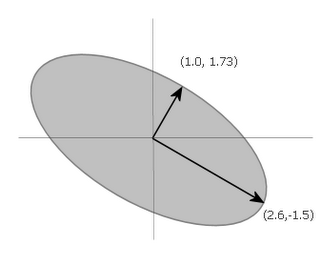The orthogonal vectors shown are the principal axes of the ellipse (semimajor axis and semiminor axis). After considering these components, let's return again to our equation:

A*V = U*S

The ellipse and its principal axes shown above give us a geometric interpretation of the result of matrix A transforming V (representing axes of the unit circle) into the ellipse whose principal axes are defined by U*S.

The columns of matrix U contain unit vectors of the axes of the ellipse. The scale matrix S contains the the length of each of those vectors along its diagonal (S[1,1] is the length of the first vector in the first column of U, S[2,2] is the length of the second vector in second column of U, etc.).

Here are some more graphics.

In 2D, an invertible (nonsingular) matrix A transforms the unit circle (or any circle) into an ellipse. The result might be circle, but a circle is an ellipse.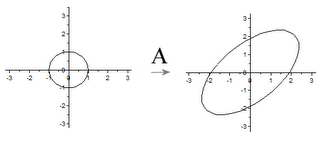In 3D, an invertible matrix A transforms the unit sphere into an ellipsoid.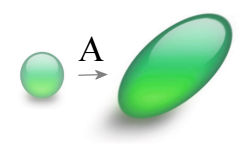Likewise, in N dimensions, an invertible matrix A transforms the unit n-sphere (hypersphere) into an n-ellipsoid (hyperellipsoid).

Viewing the SVD from the perspective of matrix A acting as a transform on the unit circle is useful in understanding the nature of the decomposition.

Update (11/18/2006): I've created a small applet to demonstrate the ideas in this post. There's an explanation of the applet in this post.

to be continued here

Wednesday, August 23, 2006

Linear Algebra for Graphics Geeks (SVD-I)

I've been meaning to write something about the Singular Value Decomposition, but I compose and compose, get too picky, leave it in my drafts folder, come back to it, don't have time. I'm doing what we all must do, if we're to get anything done: I'm going to lower my standards and just do it.

If you've done enough graphics programming, you're familiar with the use of matrices to transform vectors. Once you've got it down and learned to think in such terms, there's a huge side benefit in that you're well prepped for learning more advanced linear algebra. In fact, if you're in college and you can choose the order of the classes, I recommend taking the basic computer graphics course before linear algebra.

I believe learning matrix transformations in 2D and 3D is ideal, because it's easy to visualize and conceptualize the various operations and transformations, and almost everything you learn in the process extends to higher dimensions.

This post is written with the graphics programmer in mind. What I want to cover is bigger than a single post, so I'm going to [hopefully] post pieces as I have time. Perhaps later I can collect them into a more meaningful whole.

The subject I'm going to talk about is about the Singular Value Decomposition, or the SVD. It often isn't covered in undergraduate linear algebra courses even though it's one of the most important matrix decompositions you can learn (according to M.I.T's Gilbert Strang it's "absolutely the high point of linear algebra.").

The SVD breaks every matrix down into the product of three simpler matrices. These three simpler matrices (U, S and V) possess many useful and desirable properties. (Note the S matrix is often also called "Sigma," but it's a pain to enter sigmas in this editor, so I'll use S most of the time.)

The Singular Value Decomposition is usually written in the following form.Plainly speaking, a matrix A is factored into the product of a matrix U, a matrix S (or Sigma) and the transpose of a matrix V.

Every matrix can be factored via the SVD.

What makes the SVD extremely useful and interesting is that the matrices U, S and V have many wonderful properties, including being simple and easy to work with.

In graphics speak, U and V are rotation matrices (orthogonal matrices) and S is a scale matrix. Let's repeat that.

The SVD decomposes every matrix into rotation * scale * rotation.

This is cool all by itself, but there are also profound implications and consequences of this fact. Next time, we'll start looking into them.

I like to play with examples whenever possible. Perhaps you do too. If so, you'll find that I put a Scilab session up here.

The session contains the following examples: (1) creation of a function that returns a 2x2 rotation matrix, (2) creation of a function that returns a 2x2 scale matrix, (3) examples of calling these functions, (4) calculation of the SVD of the product of a rotation matrix and a scale matrix, (5) calculation of the product of U*S*V' and comparison with original matrix.

Scilab is an excellent and free competitor of Matlab. You can get it here.

Here's a link to the next post.

Tuesday, August 22, 2006

Arizona Sky (&c)

Now and then some strange old song emerges from the crevices of my brain. Today, Arizona Sky by China Crisis (1986). There's something in its creative quirkiness that still appeals to me. And there are Gary Daly's unmistakable nasally vocals.

This is one of those posts that serves as a verification of my existence more than anything else. The transition from summer to fall is in progress (and so, too, all the attendant adjustments).

Lately, I've become a fan of the RSS feeds on Craig's list. I've subscribed to feeds searching for items of interest. The number of matches arriving each day has surprised me.

Flash in hand, Adobe's pulling the plug on SVG. Having written an SVG rendering engine, it is for me a noteworthy obituary.

Monday, August 14, 2006

Freebie Normal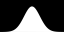The other day I had trouble finding a decent copy of the Gaussian / Normal Distribution for illustrative purposes, so I rolled my own and put them here. They're 2000 x 1000, antialiased, black and white (10-14K) PNG files that should be good enough for most purposes. Help yourself. They're free for the taking.

Sunday, August 13, 2006

Color Prints from the 1930 and 1940s

Via Digg...

"Bound for Glory," some cool color photos from the 1930s and 1940s at the Library of Congress. This one, titled Couples at a Square Dance, may have even served as the inspiration behind Brokeback Mountain.